Class 12 Work sheet practical problems on maxima and minima

5782

Mathematics Integration Level: Misc Level

Show that the surface area of a closed cuboid with square base and given volume is minimum when it is a cube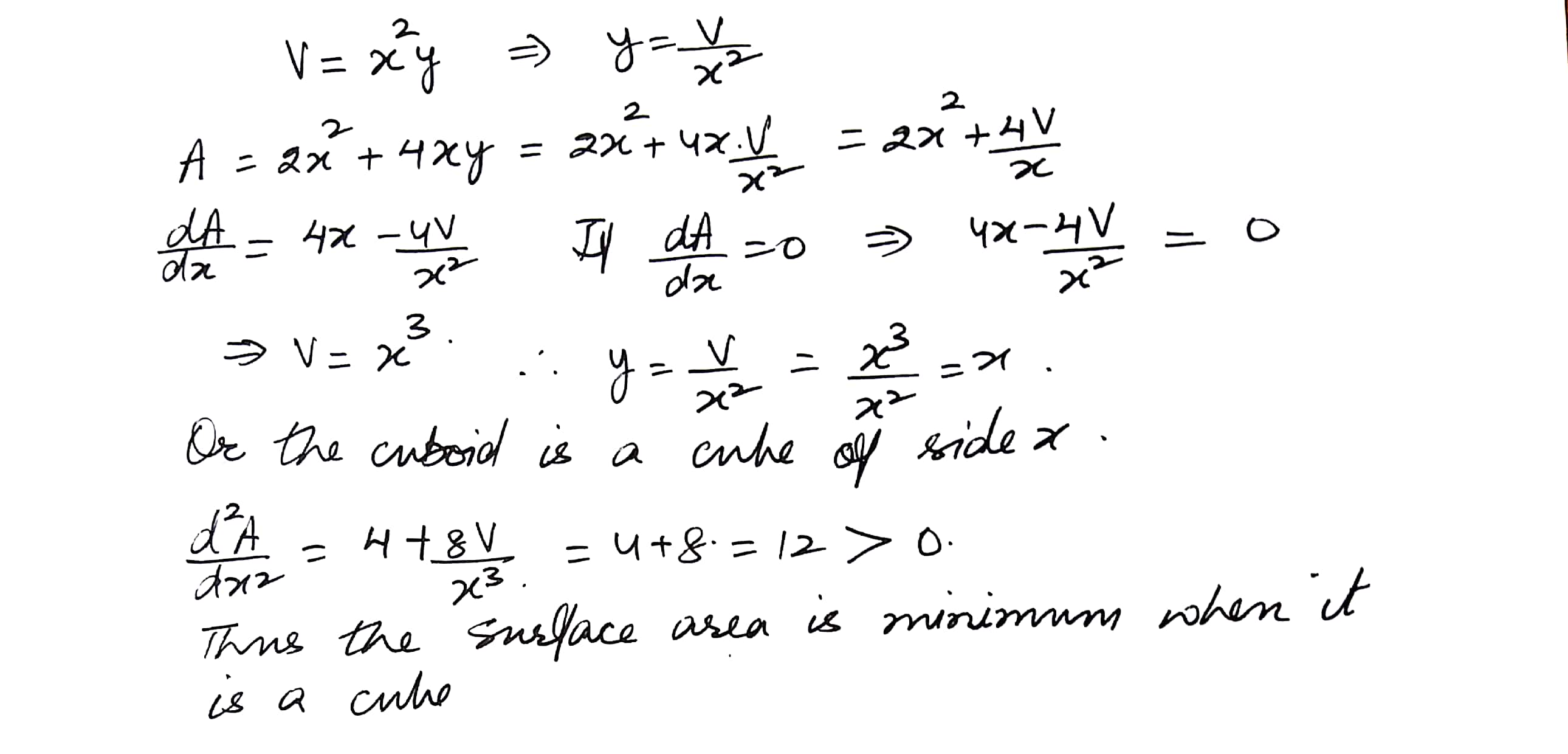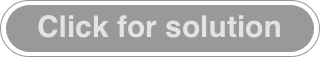5783

Mathematics Integration Level: Misc Level

An open box with a square base is to be made of given iron sheet of area 27 square meters. Show that the maximum volume of the box is 13.5 cubic meter.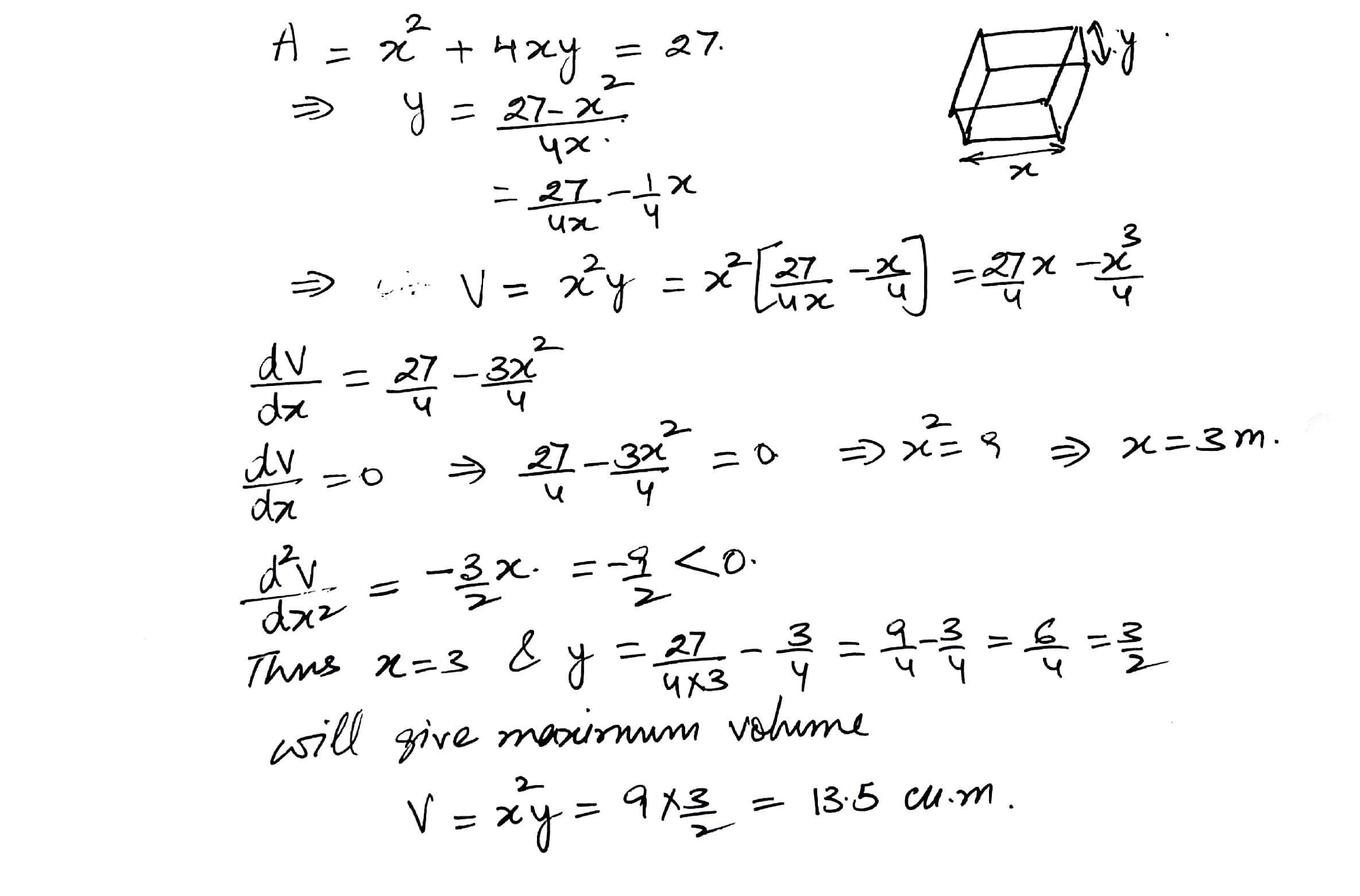5824

Mathematics Integration Level: Misc Level

A rectangular sheet of tin 45 cm by 24 cm is to be made into a box without top by cutting off squares from the corners and folding up the flaps. What should be the side of the square in order that the volume of the box is maximum?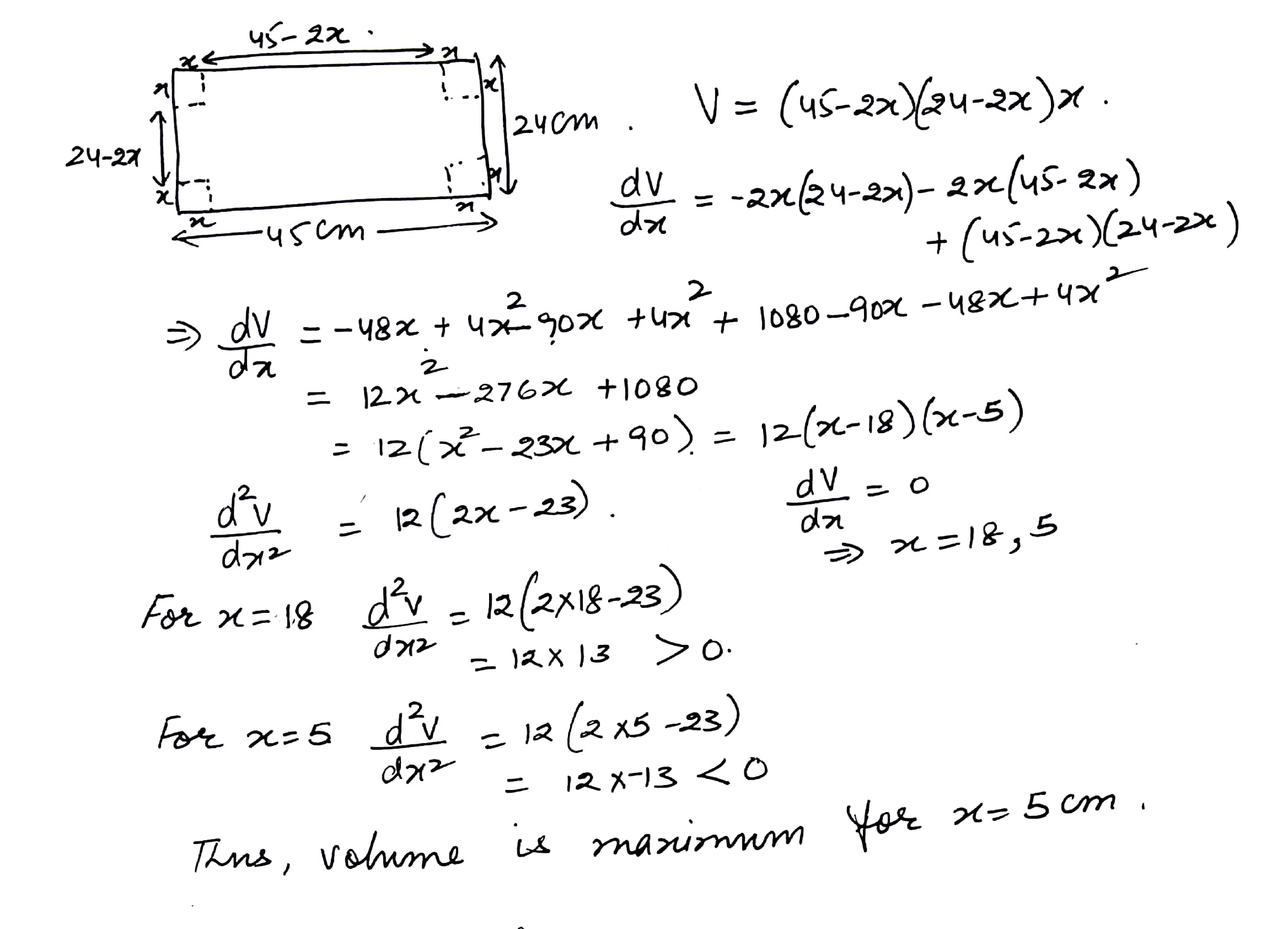5825

Mathematics Integration Level: Misc Level

A closed right circular cylinder has a volume of 2156 cubic cm. What will be the radius of its base so that its total surface area is minimum? Find the height of the cylinder when its total surface is minimum. Take =22/7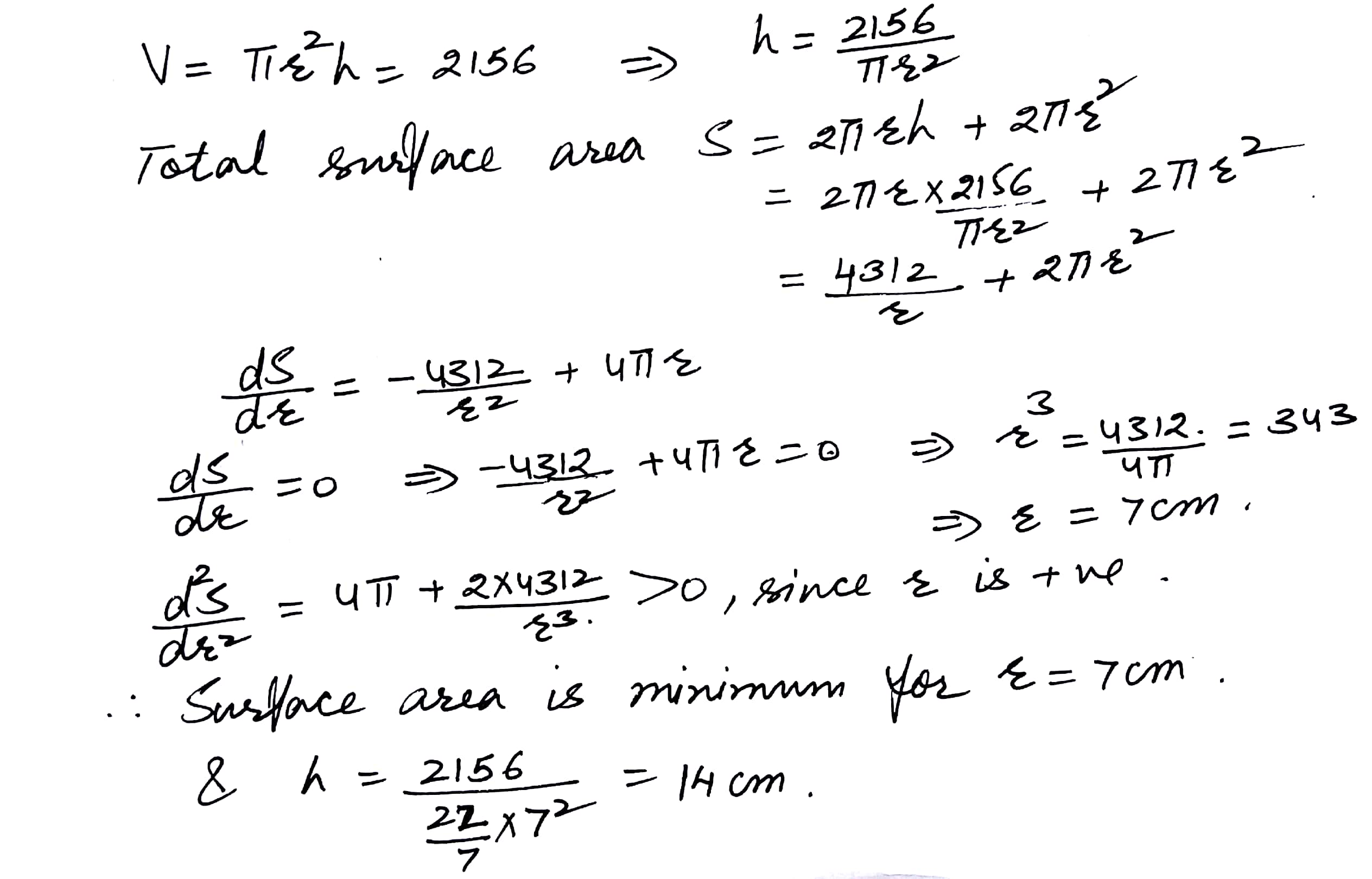5826

Mathematics Integration Level: Misc Level

Find the maximum volume of the cylinder which can be insrcribed in a sphere of radius cm. (Leave the answer in terms of )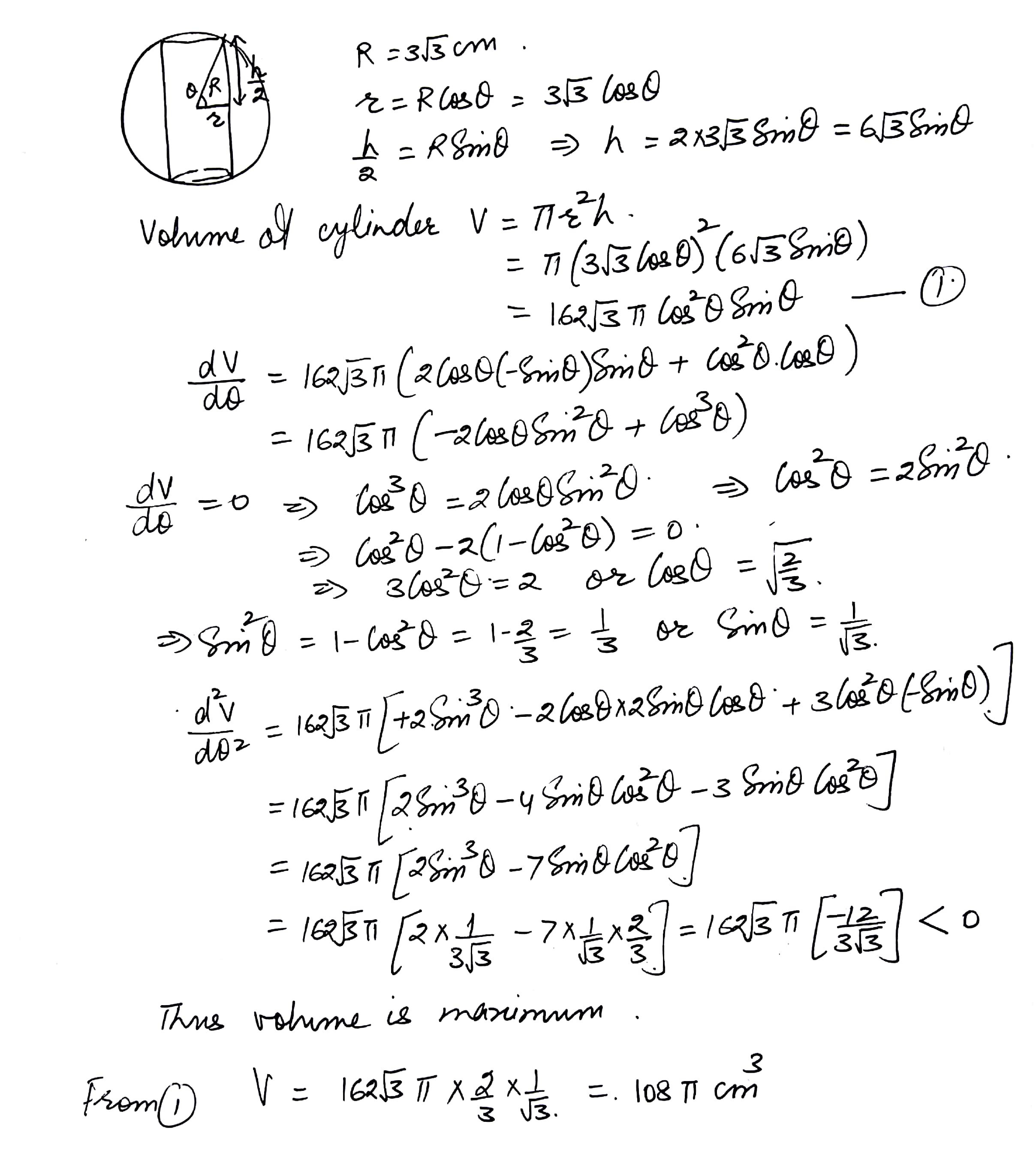5827

Mathematics Integration Level: Misc Level

Show that the semi-vertical angle of the right circular cone of given total surface area and maximum volume is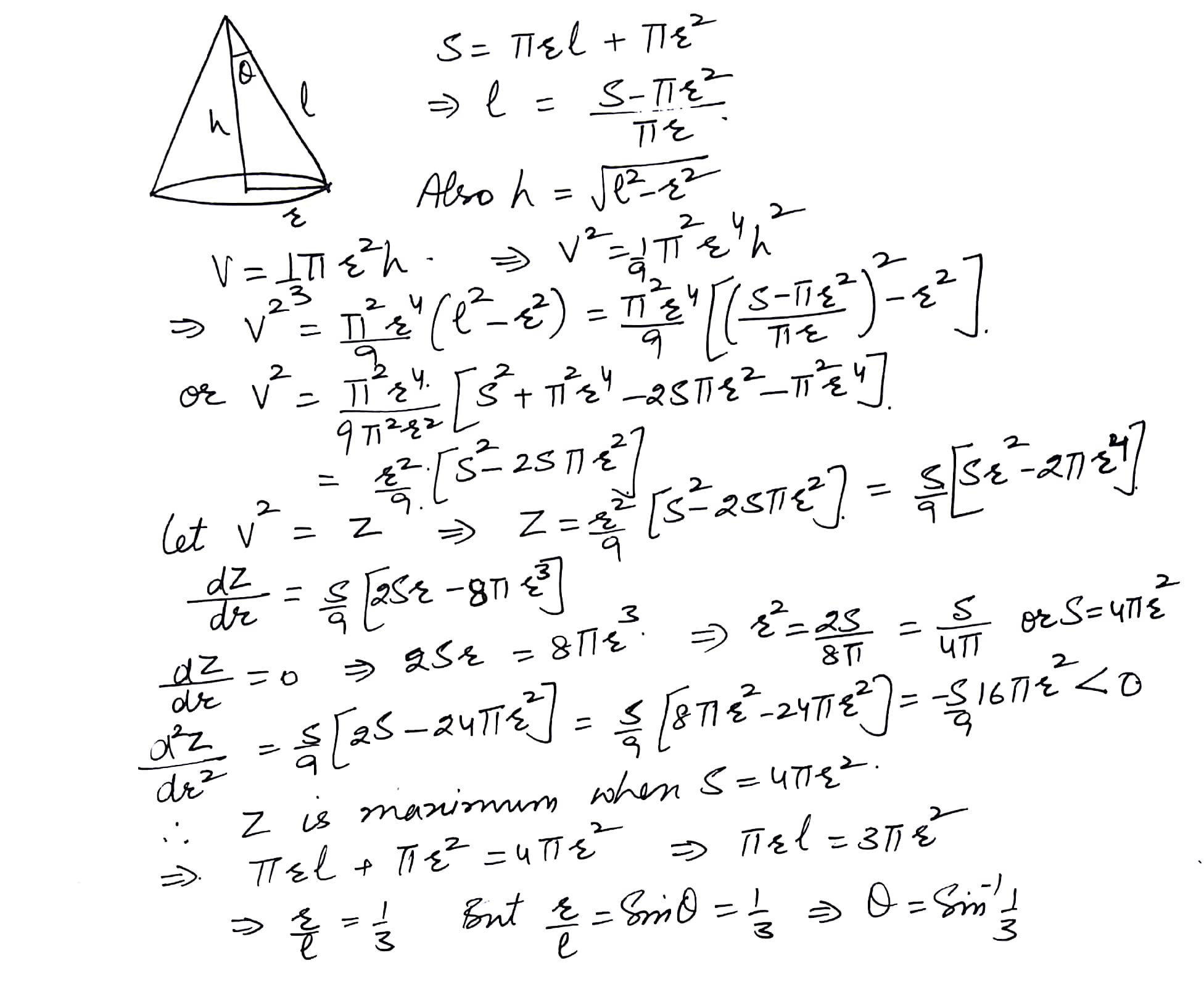5828

Mathematics Integration Level: Misc Level

An open box with a square base is to be made out of a given quantity of cardboard whose area is c2 square units. Show that the maximum volume of the box is cubic units.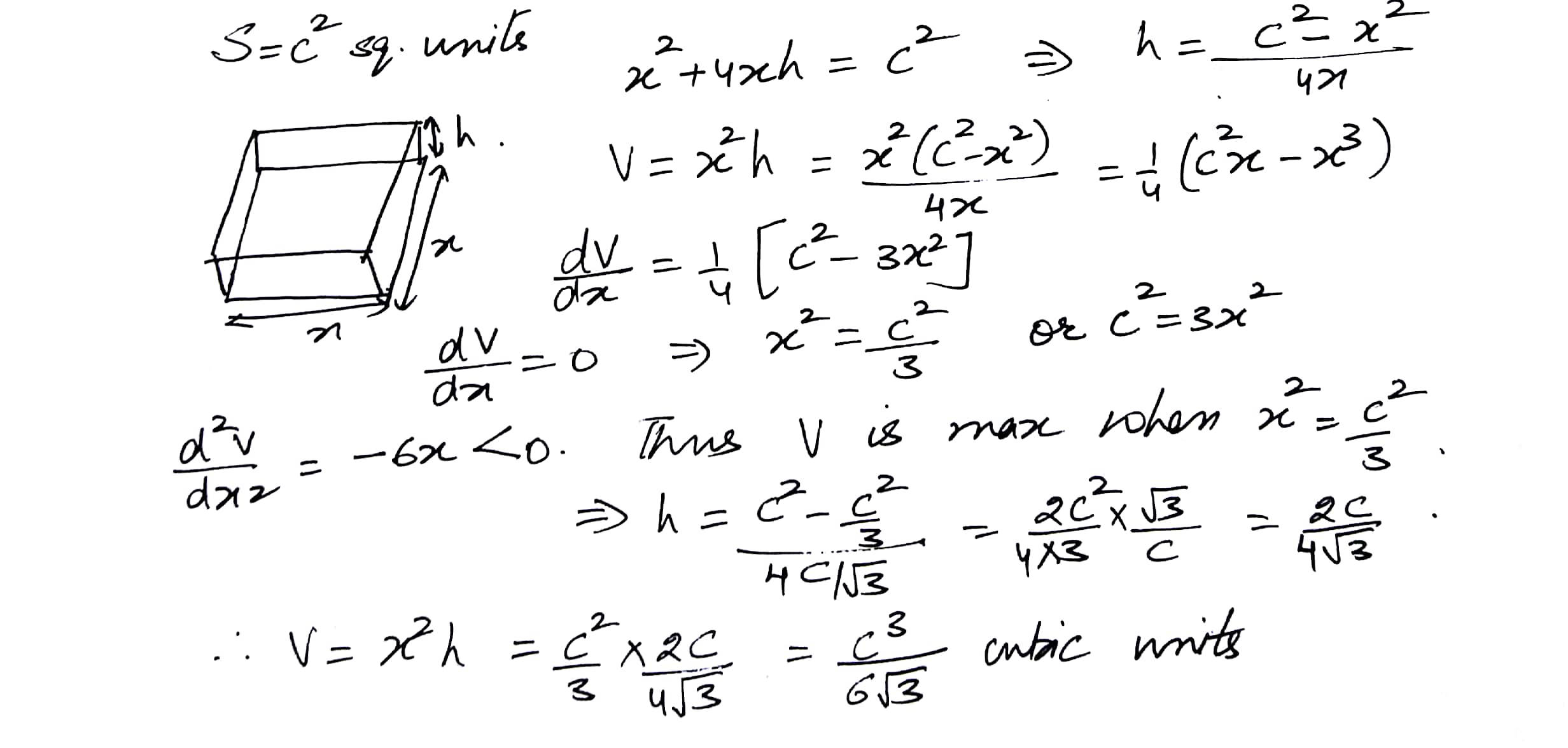Displaying 11-17 of 17 results.